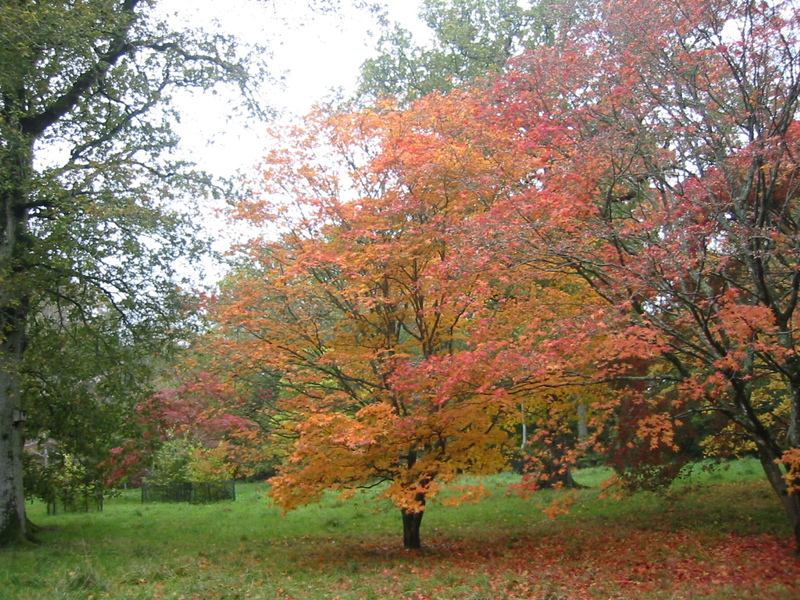# How many leaves are there on a tree?### The Basic Idea

Estimate the number of leaves on a tree and factor this up to estimate how many leaves might be found in an area such as your school field or local park.

• This activity can be carried out from when the leaves appear in Spring up until before they fall in Autumn.
• Take out your group to explore the trees growing in your school grounds, local park, woodland or green space. Get your group to have a quick guess at how many leaves might grow on an individual tree and write these down.
• Then send groups off with their pads, calculators and tally counters (if you have them). Ask them to count how many leaves they can find on a number of branches of varying size. Ask them to calculate an average from these. Ask them to count how many branches their tree has. Ask them to estimate from this the number of leaves on their tree.
• Get your groups back together and compare figures. How did their initial guesses vary from their calculated estimates? How much did the number of leaves vary from one tree to another?

### How to take it even further or make it more challenging

Introduce the idea that a gardener sometimes has to collect up all the leaves from these spaces.

If you have not estimated the number of leaves on all the trees present in a given area then calculate an average number of leaves for a tree in the space you are in and multiply this by the number of trees present.

Using your estimate of the number of leaves in your area explore how long it might take for someone to clear all these up.

Consider how many leaves (on average) fit on a shovel?

How long might it take to gather up one shovel full on leaves?

Divide the estimated total number of leaves in your area, by the estimated number of leaves that fit on a shovel.

Multiply the time taken to collect one shovel by the total number of shovels and hey presto – that’s how long it will take to clear them all up.